### 13.1.1.2 Parametric constraints

The parametric way of expressing velocity constraints gives a different interpretation to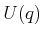. Rather than directly corresponding to a velocity, each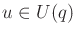is interpreted as an abstract action vector. The set of allowable velocities is then obtained through a function that maps an action vector into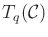. This yields the configuration transition equation (or system)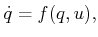(13.2)

in which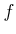is a continuous-time version of the state transition function that was developed in Section 2.1. Note that (13.2) actually representsscalar equations, in whichis the dimension of. The system will nevertheless be referred to as a single equation in the vector sense. Usually,is fixed for all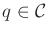. This will be assumed unless otherwise stated. In this case, the fixed action set is denoted as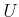.

There are two interesting ways to interpret (13.2):

1. Subspace of the tangent space: If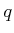is fixed, thenmaps frominto. This parameterizes the set of allowable velocities atbecause a velocity vector,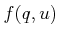, is obtained for every.
2. Vector field: Ifis fixed, thencan be considered as a function that maps eachinto. This means thatdefines a vector field overfor every fixed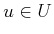.

Example 13..1 (Two Interpetations of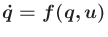)   Suppose that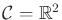, which yields a two-dimensional velocity vector space at every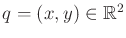. Let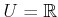, andbe defined as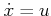and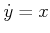.

To obtain the first interpretation of, hold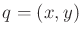fixed; for each, a velocity vector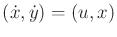is obtained. The set of all allowable velocity vectors atis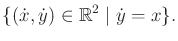(13.3)

Suppose that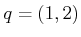. In this case, any vector of the form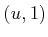for any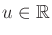is allowable.

To obtain the second interpretation, holdfixed. For example, let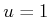. The vector field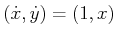over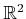is obtained.It is important to specifywhen defining the configuration transition equation. We previously allowed, but discouraged, the action set to depend on. Any differential constraints expressed asfor anycan be alternatively expressed as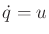by defining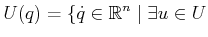such that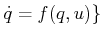(13.4)

for each. In this definition,is not necessarily a subset of. It is usually more convenient, however, to use the formand keep the samefor all. The common interpretation ofis that it is a set of fixed actions that can be applied from any point in the C-space.

In the context of ordinary motion planning, a configuration transition equation did not need to be specifically mentioned. This issue was discussed in Section 8.4. Provided thatcontains an open subset that contains the origin, motion in any direction is allowed. The configuration transition equation for basic motion planning was simply. Since this does not impose constraints on the direction, it was not explicitly mentioned. For the coming models in this chapter, constraints will be imposed on the velocities that restrict the possible directions. This requires planning algorithms that handle differential models and is the subject of Chapter 14.

Steven M LaValle 2020-08-14